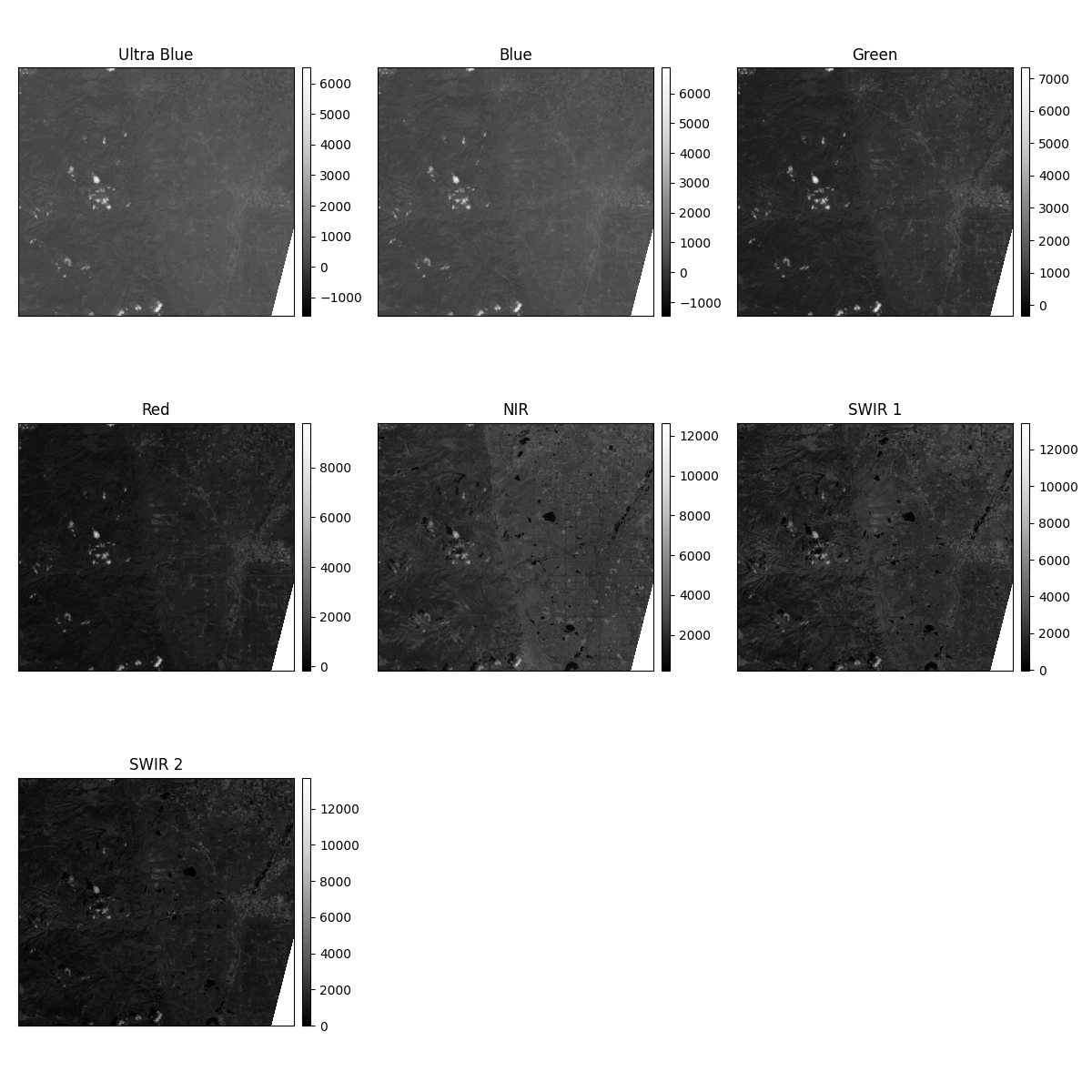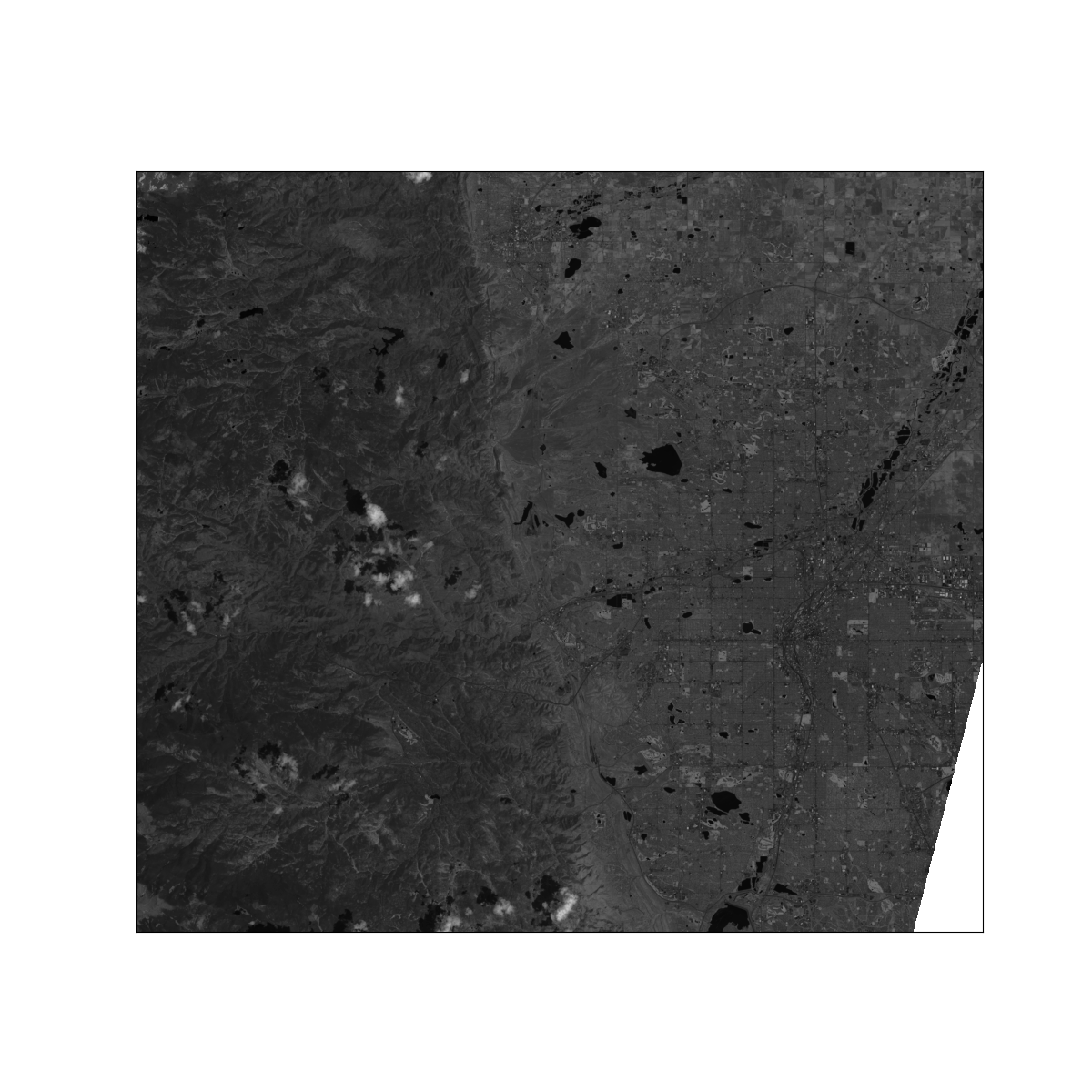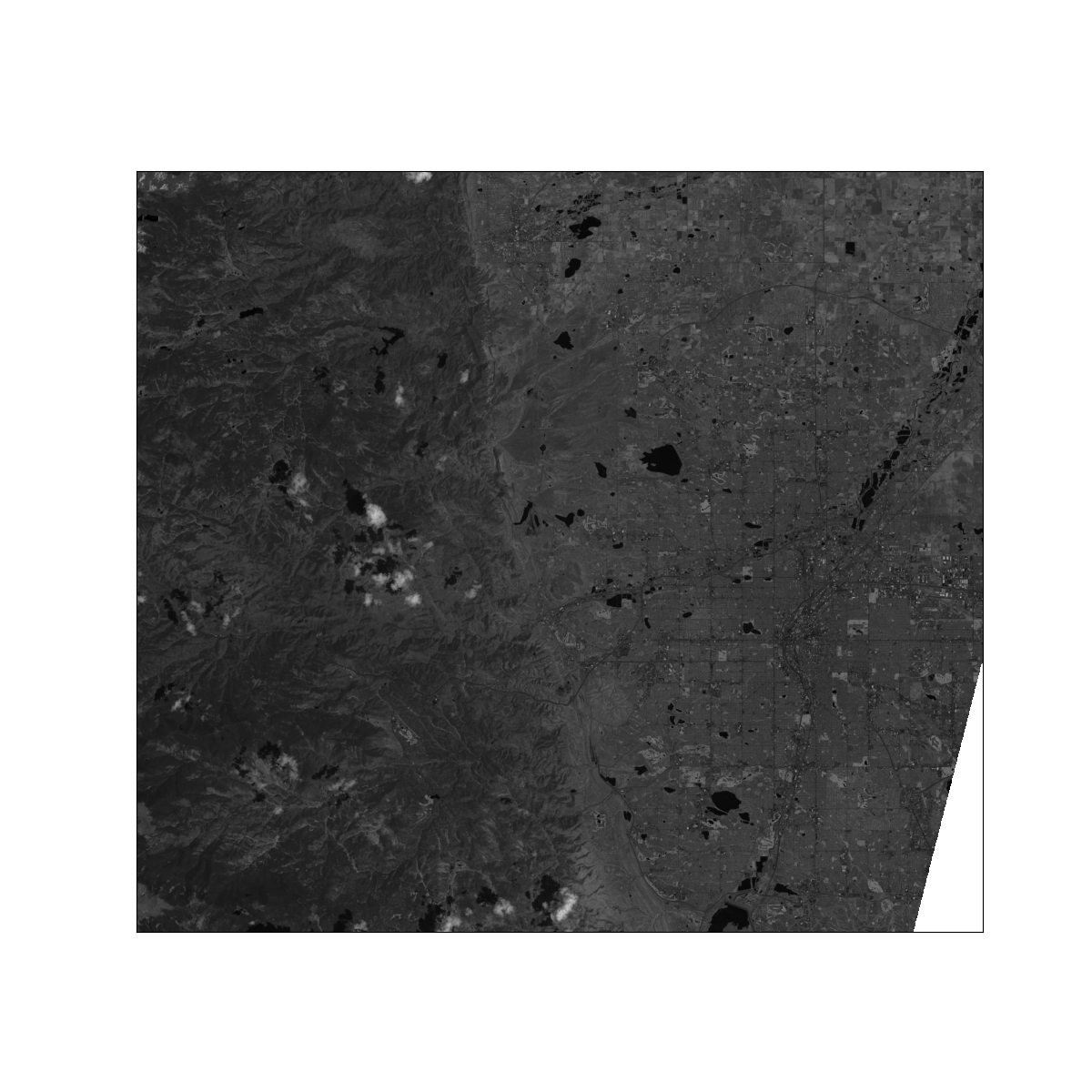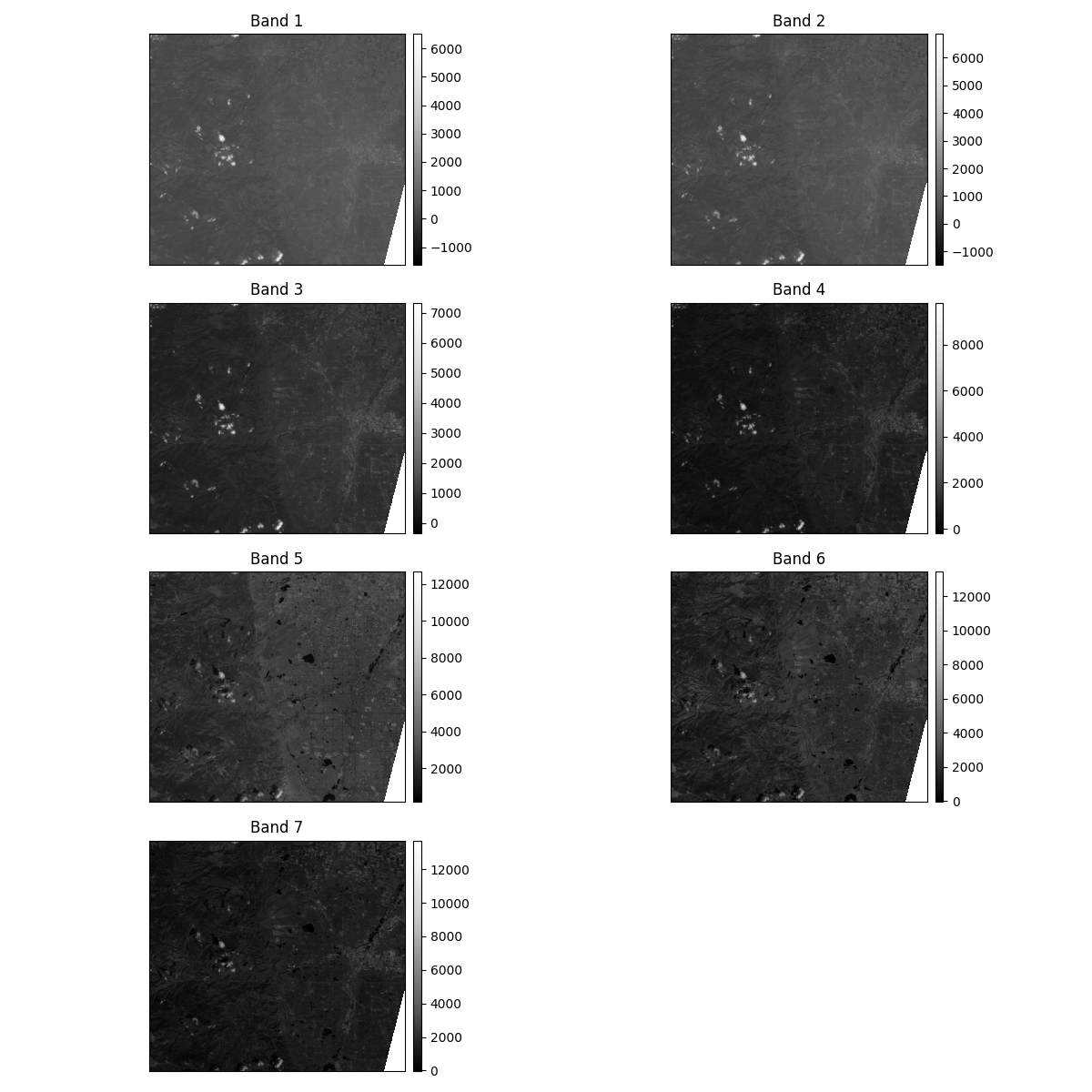Plot Bands of Satellite Imagery with EarthPy

Learn how to use the EarthPy plot_bands() function to quickly plot raster bands for an image. Plot_bands() can be used to both plot many bands with one command with custom titles and legends OR to plot a single raster layer with (or without) a legend.

Plot Raster Data Layers Using EarthPy

Note

The examples below will show you how to use the plot_bands() function to plot individual raster layers in images using python. To plot rgb data, read help documentation related to ep.plot_rgb().

In this vignette, you will use Landsat 8 data. To begin, you will create a stack of bands using Landsat 8 data. You will then plot the raster layers.

Import Packages

In order to use the plot_bands() function with Landsat 8 data, the following packages need to be imported.

import os
from glob import glob
import matplotlib.pyplot as plt
import earthpy as et
import earthpy.spatial as es
import earthpy.plot as ep

Import Example Data

To get started, make sure your directory is set. Then, create a stack from all of the Landsat .tif files (one per band).

# Get data for example
data = et.data.get_data("vignette-landsat")

# Set working directory
os.chdir(os.path.join(et.io.HOME, "earth-analytics"))

# Stack the Landsat 8 bands
# This creates a numpy array with each "layer" representing a single band
# You can use the nodata= parameter to mask nodata values
landsat_path = glob(
os.path.join(
"data",
"vignette-landsat",
"LC08_L1TP_034032_20160621_20170221_01_T1_sr_band*_crop.tif",
)
)
landsat_path.sort()
array_stack, meta_data = es.stack(landsat_path, nodata=-9999)

Out:

Extracted output to /home/docs/earth-analytics/data/vignette-landsat/.

Plot All Bands in a Stack

When you give ep.plot_bands() a three dimensional numpy array, it will plot all layers in the numpy array. You can create unique titles for each image by providing a list of titles using the title= parameter. The list must contain the same number of strings as there are bands in the stack.

titles = ["Ultra Blue", "Blue", "Green", "Red", "NIR", "SWIR 1", "SWIR 2"]
# sphinx_gallery_thumbnail_number = 1
ep.plot_bands(array_stack, title=titles)
plt.show()Plot One Band in a Stack

If you give ep.plot_bands() a one dimensional numpy array, it will only plot that single band. You can turn off the colorbar using the cbar parameter (cbar=False).

ep.plot_bands(array_stack, cbar=False)
plt.show()Turn On Scaling

ep.plot_bands() does not scale imagery to a 0-255 scale by default. However, often this range of values makes it easier for matplotlib to plot the data. To turn on scaling, set the scale parameter to True. Below you plot band 5 of the satellite imagery with scaling turned on in order to see the data without the values being modified.

ep.plot_bands(array_stack, cbar=False, scale=True)
plt.show()Adjust the Number of Columns for a Multi Band Plot

The number of columns used while plotting multiple bands can be changed in order to change the arrangement of the images overall.

ep.plot_bands(array_stack, cols=2)
plt.show()Total running time of the script: ( 0 minutes 10.991 seconds)

Gallery generated by Sphinx-Gallery